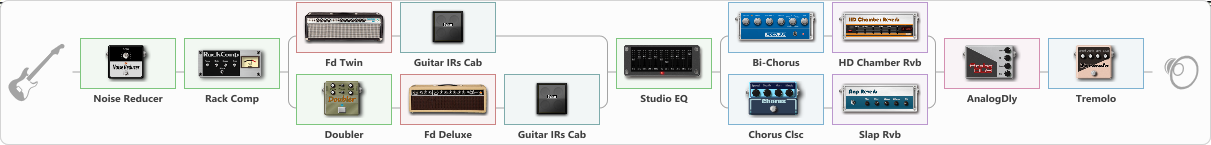# Jazz-rhythm with touch of chorus and tremolo

Discussion in 'ToneLib-GFX presets' started by chartobani, Jul 30, 2022.

1. Jazz-rhythm with touch of chorus and tremolo

Preset name: Chorus touch Tremolo touch

Bebop rhythm guitar sound - use neck pickup with a lots of gain and a light touch

Effects chain:Effect: "Noise Reducer" (Dynamics / Filter), active - "yes"
{
"Sens" = 28
"Mode" = Soft
}

Effect: "Rack Comp" (Dynamics / Filter), active - "yes"
{
"Threshold (dB)" = -34
"Ratio" = 8
"Attack" = Fast
"Release (ms)" = 694
"Knee" = 0
"Level (dB)" = 8
}

Effect: "Splitter" (Dynamics / Filter)
{
"A-Bypass" = Off
"A-Pan" = -51
"A-Level" = 55
"B-Bypass" = Off
"B-Pan" = 52
"B-Level" = 63
"Width" = 0

'A' branch:
{

Effect: "Fd Twin" (Amp simulators), active - "yes"
{
"Gain" = 49
"Bass" = 48
"Middle" = 52
"Treble" = 35
"Presence" = 28
"Master" = 70
"Level (dB)" = 0
}

Effect: "Guitar IRs Cab" (Cabinets), active - "yes"
{
"Model" = Fender Twin Rev (2x12")
"Mic Position" = Middle
"Mic Distance" = Near
"Low Cut (Hz)" = 60
"Hi Cut (kHz)" = 20.0
"Mix" = 85
"Level (dB)" = 0
}
}
'B' branch:
{

Effect: "Doubler" (Dynamics / Filter), active - "yes"
{
"A-Dly" = 75
"A-Inv" = On
"A-Level" = 55
"B-Dly" = 64
"B-Inv" = Off
"B-Level" = 55
}

Effect: "Fd Deluxe" (Amp simulators), active - "yes"
{
"Gain" = 29
"Bass" = 62
"Middle" = 61
"Treble" = 59
"Presence" = 36
"Master" = 53
"Level (dB)" = 0
}

Effect: "Guitar IRs Cab" (Cabinets), active - "yes"
{
"Model" = Vox AC30 (2x12")
"Mic Position" = Middle
"Mic Distance" = Near
"Low Cut (Hz)" = 60
"Hi Cut (kHz)" = 20.0
"Mix" = 90
"Level (dB)" = 0
}
}
}

Effect: "Studio EQ" (Dynamics / Filter), active - "yes"
{
"31 Hz" = -8
"62 Hz" = -3
"125 Hz" = 0
"250 Hz" = 3
"500 Hz" = 4
"1 kHz" = -1
"2 kHz" = -5
"4 kHz" = -6
"8 kHz" = -5
"16 kHz" = -4
"Level (dB)" = 10
}

Effect: "Splitter" (Dynamics / Filter)
{
"A-Bypass" = Off
"A-Pan" = 20
"A-Level" = 55
"B-Bypass" = Off
"B-Pan" = -20
"B-Level" = 55
"Width" = 0

'A' branch:
{

Effect: "Bi-Chorus" (Modulation / Sfx), active - "yes"
{
"Speed A" = 3.5
"Speed B" = 3.4
"Depth" = 43
"Reso" = 0
"Mode" = Parallel
"Mix" = 8
}

Effect: "HD Chamber Rvb" (Reverberation), active - "yes"
{
"Time" = 7.6
"PreLPF" = 79
"PreDelay" = 61
"HiDamp" = 21
"LoGain" = -2.1
"Mix" = 14
}
}
'B' branch:
{

Effect: "Chorus Clsc" (Modulation / Sfx), active - "yes"
{
"Speed" = 4.0
"Depth" = 11
"Center" = 3.0
"Mode" = Stereo
}

Effect: "Slap Rvb" (Reverberation), active - "yes"
{
"Time" = 7.3
"PreDelay" = 0
"LoDamp" = 18
"HiDamp" = 40
"Mix" = 9
}
}
}

Effect: "AnalogDly" (Delay), active - "yes"
{
"Time" = 100
"Feedback" = 0
"Tone" = 0
"Mix" = 13
}

Effect: "Tremolo" (Modulation / Sfx), active - "yes"
{
"Speed" = 6.0
"Depth" = 13
"Level" = 33
}

Note: You will need to download and install the ToneLib-GFX software to use the preset.

File size:
1.7 KB
Views:
1,802